# Two machines produce metal parts. The mean of the weight of these parts is of interest. The following data have been collected Machine 1 N 30 x(dash) = 0.984 σ 3.67 Machine 2 n 35 x (dash) 0.907 σ 3.1...

Two machines produce metal parts. The mean of the weight of these parts is of interest. The following data have been collected

Machine 1

N 30

x(dash) = 0.984

σ 3.67

Machine 2

n 35

x (dash) 0.907

σ 3.11

a. Do both machines produce parts with a statistical same mean weight? State your null and alternative hypothesis, and use a 1% level of significance.

b. If we want the confidence interval to have a half width of 0.05, how many pieces should be collected?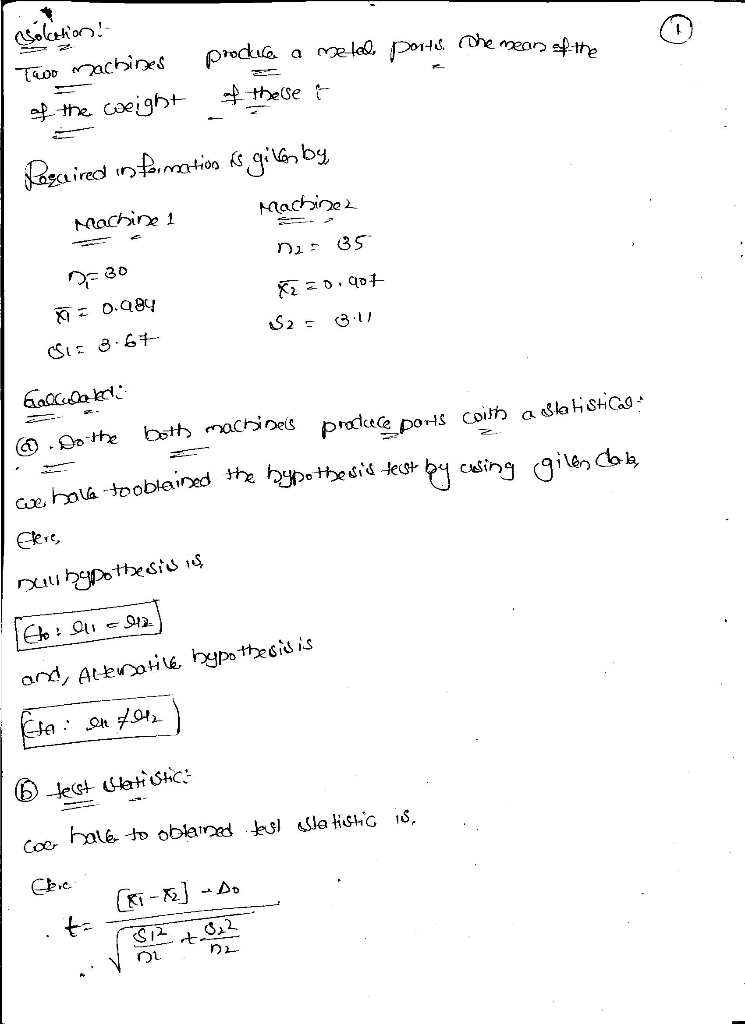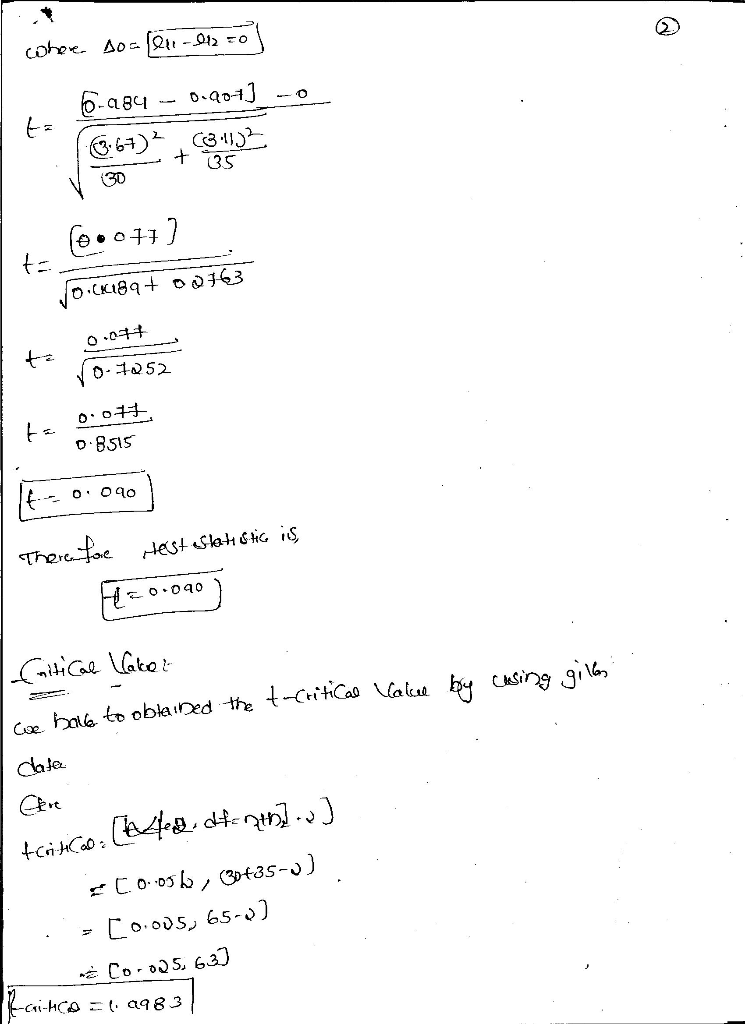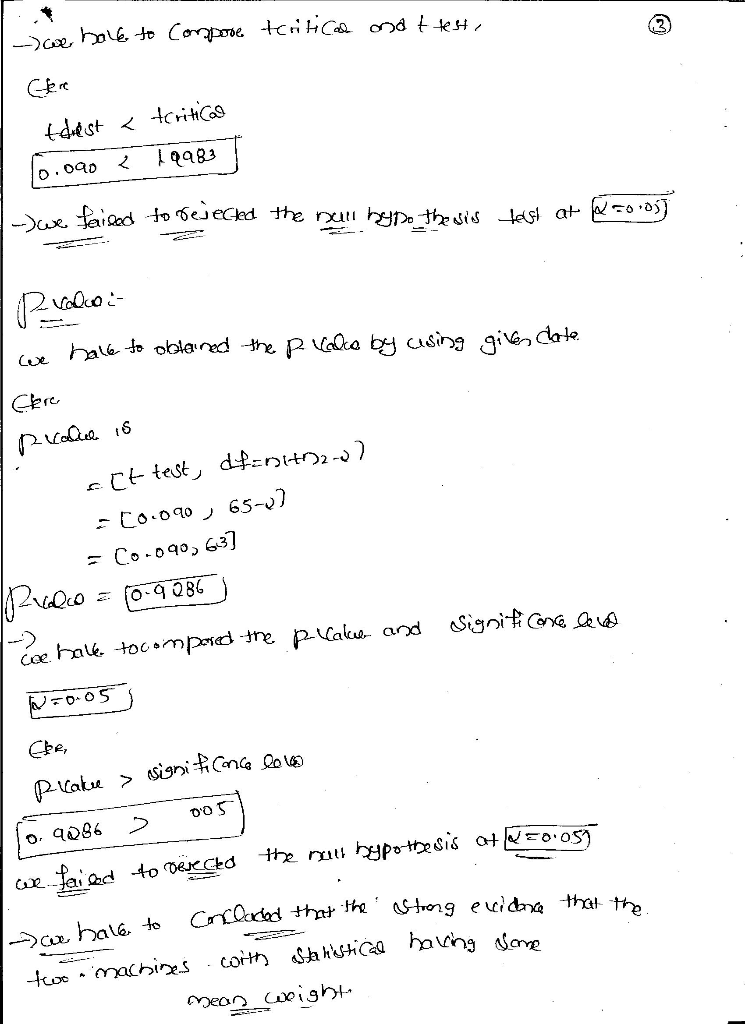Due to insufficieint time , i will not answer question (b) .

Thank you

##### Add Answer of: Two machines produce metal parts. The mean of the weight of these parts is of interest. The following data have been collected Machine 1 N 30 x(dash) = 0.984 σ 3.67 Machine 2 n 35 x (dash) 0.907 σ 3.1...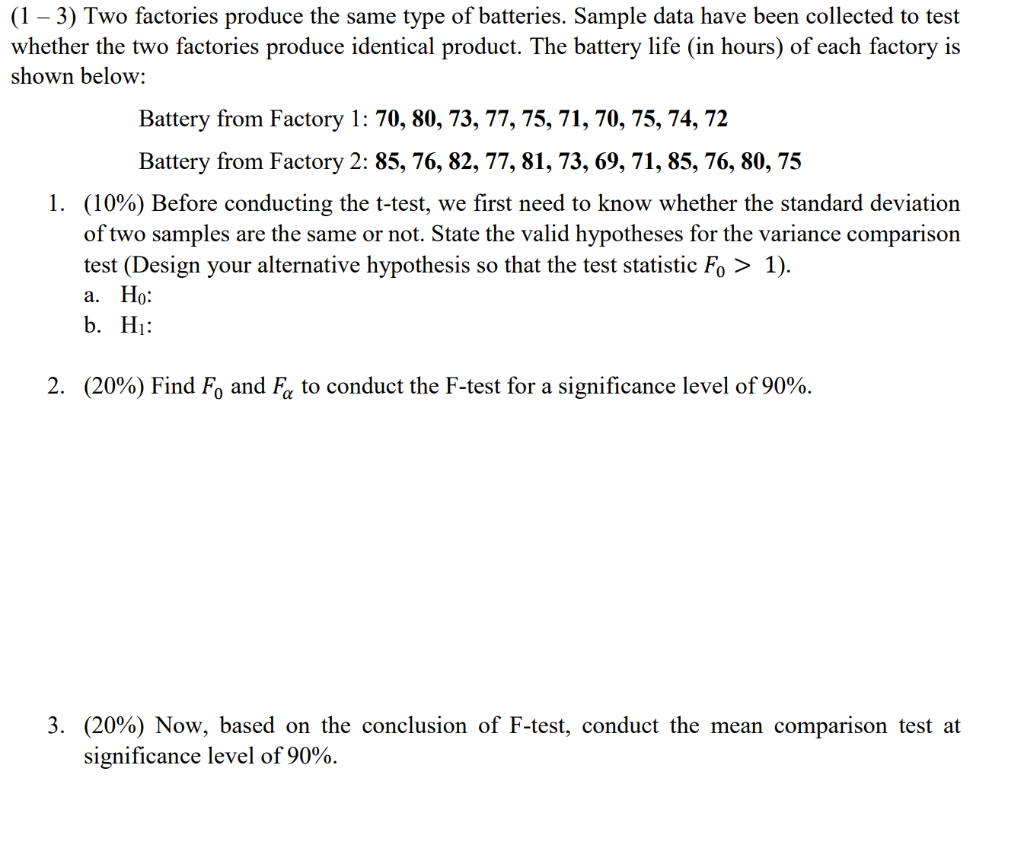(1 - 3) Two factories produce the same type of batteries. Sample data have been collected to test whether the two factories produce identical product. The battery life (in hours) of each factory is shown below: Battery from Factory 1: 70, 80, 73, 77,75,71,70, 75, 74, 72 Battery from Factory 2: 85, 76, 82, 77, 81, 73, 69, 71,85, 76, 80, 75 (10%) Before conducting the t-test, we first need to know whether the standard deviation of two samples are...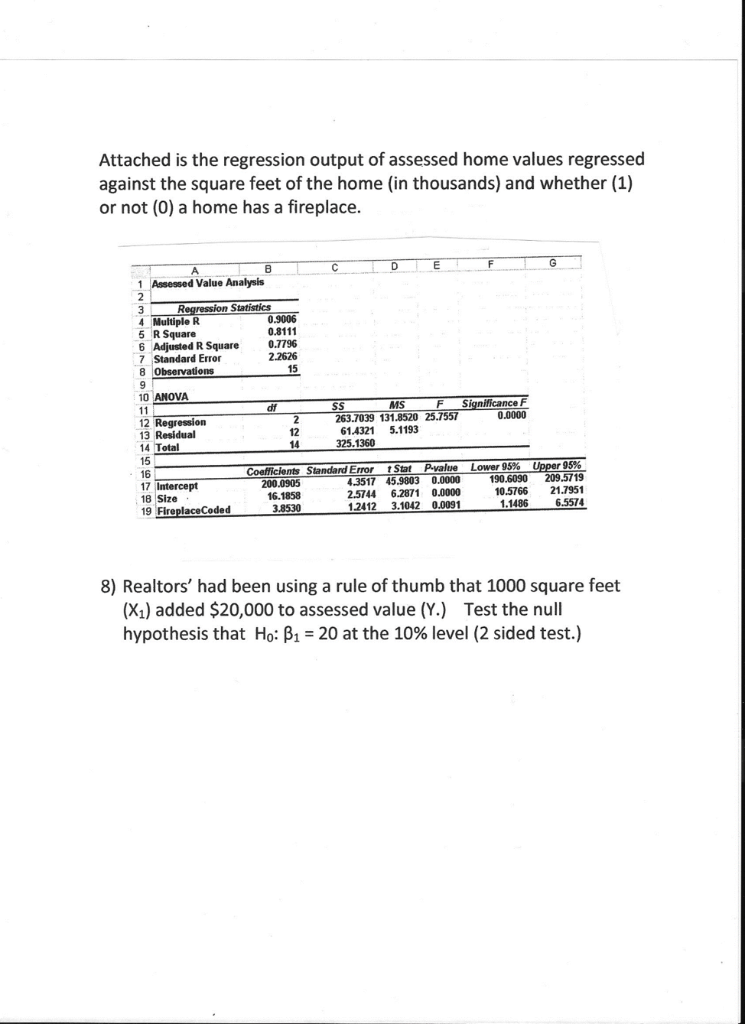The following ANOVA model is for a multiple regression model with two independent variables: Degrees of            Sum of                 Mean Source           Freedom            Squares                Squares       F       Regression            2                    60 Error                   18                 120 Total                   20                 180 Determine the Regression Mean Square (MSR): Determine the Mean Square Error (MSE): Compute the overall Fstat test statistic. Is the Fstat significant at the 0.05 level? A linear regression was run on auto sales relative to consumer income. The Regression Sum of Squares (SSR) was 360 and...